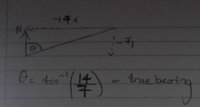# Bearings Question

#### S_100

##### New member
Q is such A guard at O monitors the movement of a boat.

At 10:00 the boat is (8i-5j) km relative to O

At 12:50 the boat is at the point (-6i-12j) km relative to O

The motion of the boat is modelled as a particle moving at a constant speed on a straight line.

(a) Calculate the bearing on which the boat is moving.

For question (a), I 1. calculated the difference in position vectors 1. -6-8 i + -12+5 j = -14i -7j

I then calculated tan-1(14/7) = 64.434... deg

would this be correct? I am aware that this is not the velocity vector and that I would have to divide both magnitudes by the time elapsed between the two position vectors, that is 2 hr:50 mins = (17/6) hr = 2 .833333... hr

but surely it is just a scale factor so my answer angle would be the same?

(b) calculate the speed of the boat giving your answer in km hr-1

Finding the velocity vector
-(14/2.83333) i - (7/2.83333) j

2. I square root [ (14/2.83333)2+ (7/2.83333)2 ]

Are my workings and method correct?

Thank you,

#### Harry_the_cat

##### Elite Member
Have you drawn a diagram? arctan(14/7)=63.435 degrees approx.
But is this the bearing from its initial position to its final position?
Are you going to give your answer as a compass bearing or true bearing (from North)?

For (b) What is the distance travelled? Then find the speed by calculating distance/time taken.

Let us know what you get.

#### S_100

##### New member
Are you going to give your answer as a compass bearing or true bearing (from North)?
Hi Harry the Cat,

Yes I am confused on, whether I should give a bearing from initial to final position or as a true bearing from North. I think the latter bearing would be needed in (a) so I would recognise that the supplementary angle is 180-63.434..., and then do 360-(180-63.434...)= 243.4349.... degrees
Would this be correct?

for (b) distance travelled= displacement =√(142+7 )=7√5 km

and then 7√5 m/ 2.83333333.... hours = 5.52 km hr-1. to 3sf

Out of interest why isn't √(142+72 /2.833332) a correct way of doing the latter question too, do not both calculate constant speed? Or is just the former way calculated for avg/constant speed?

#### Harry_the_cat

##### Elite Member
Hi Harry the Cat,

Yes I am confused on, whether I should give a bearing from initial to final position or as a true bearing from North. I think the latter bearing would be needed in (a) so I would recognise that the supplementary angle is 180-63.434..., and then do 360-(180-63.434...)= 243.4349.... degrees
Would this be correct?

for (b) distance travelled= displacement =√(142+7 )=7√5 km

and then 7√5 m/ 2.83333333.... hours = 5.52 km hr-1. to 3sf

Out of interest why isn't √(142+72 /2.833332) a correct way of doing the latter question too, do not both calculate constant speed? Or is just the former way calculated for avg/constant speed?
A) correct
B) both methods are correct

•S_100

#### S_100

##### New member
direction of motion from initial to final position would be $$\displaystyle \arctan(1/2) + 180^\circ \approx 207^\circ$$ relative to the positive x-axis. Therefore, the direction relative to true north would be on a bearing of $$\displaystyle 243^\circ$$

speed = $$\displaystyle \dfrac{7\sqrt{5} \, km}{17/6 \, hrs} \approx 5.5 \, km/hr$$

View attachment 25240Wait I don't understand how true bearing is 207 to 3s.f. Surely to calculate true north bearing - that runs clockwise to the line of motion, I could calculate the angle subtended by the North line and the drawn line of motion, as seen above.

#### skeeter

##### Elite Member
Wait I don't understand how true bearing is 207 to 3s.f. Surely to calculate true north bearing - that runs clockwise to the line of motion, I could calculate the angle subtended by the North line and the drawn line of motion, as seen above.
Reread my post. 207 is not a true bearing ... what is the calculator derived 207 degrees relative to?

#### S_100

##### New member
Reread my post. 207 is not a true bearing ... what is the calculator derived 207 degrees relative to?
Ah my bad 207 from initial to final position relative to the positive x-axis, sorry, my question was supposed to read how is the true bearing 243 if I can calculate true bearing as seen in my image above?

#### S_100

##### New member
I think we're on different pages on which position vector the true north is calculated from. I chose the leftmost point, that is, at -6i-12j, which if I am not wrong would be arctan(2). Why have you calculated true north at the initial point at 10:00 and not at 12:50?

#### Harry_the_cat

##### Elite Member
I think we're on different pages on which position vector the true north is calculated from. I chose the leftmost point, that is, at -6i-12j, which if I am not wrong would be arctan(2). Why have you calculated true north at the initial point at 10:00 and not at 12:50?
The boat is moving FROM the rightmost point TO the leftmost. The direction of travel is always measured from the point you are travelling FROM.